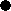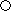# M-MIND

This game is called "Mastermind" in English. This version uses the 1-8 numbers and they aren't repeated in the hidden combination.
You will also need a table like the one below to play the game.10
9
8
7
6
5
4
3
2
1
• your goal is to guess the combination of 5 pegs (colors are represented by numbers 1-8)
• enter your tip as a five-digit number (e.g. enter "75435" to try 7,5,4,3,5)
• program then tells you the feedback as two-digit number (e.g. when 1 peg is at correct positions and 2 at wrong position, it outputs "12")

## Source code (423 B)

``` T= X= A= B= LABEL 1 R=INT 8RANDOM+1 IF .1<=FPART (.1B/10^R GOTO 1 B=B+10^R T=T+R10^X X=X+1 IF X≠5 GOTO 1 N=RANDOM LABEL 5 N=>RAND T=T/RANDOM B=B/RANDOM LABEL 2 CLRT PRINT"TIP INPUT P IF INT log P≠4 GOTO 2 N=>RAND T=TRANDOM B=BRANDOM X= I= J= A=A+1 LABEL 3 R=10FPART (.1INT (P10^-X Q=10FPART (.1INT (T10^-X I=I+INT (1-.1ABS (R-Q J=J+INT (10FPART (.1B/10^R X=X+1 IF X≠5 GOTO 3 CLRT IF I=5 GOTO 4 PRINT"CORRECT,MISPL. O=9I+J PRINT O WAIT IF A<10 GOTO 5 CLRT PRINT"THE END! PRINT T END LABEL 4 PRINT"WELL DONE! PRINT A ```